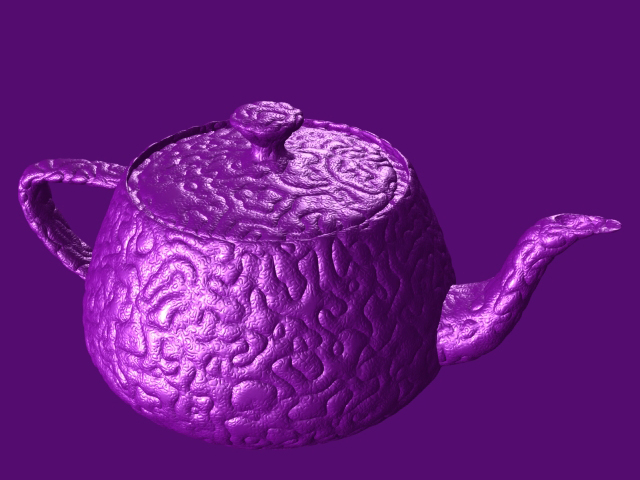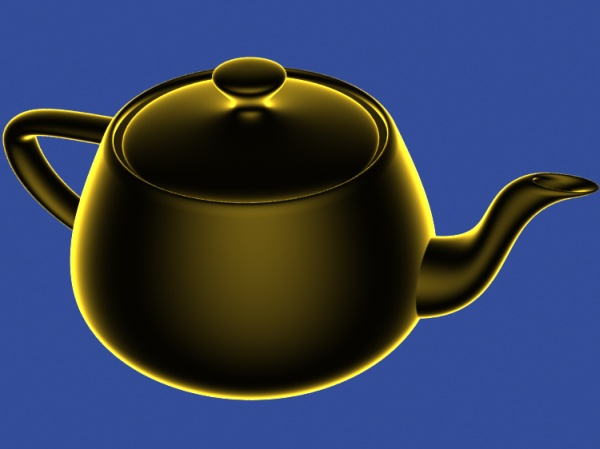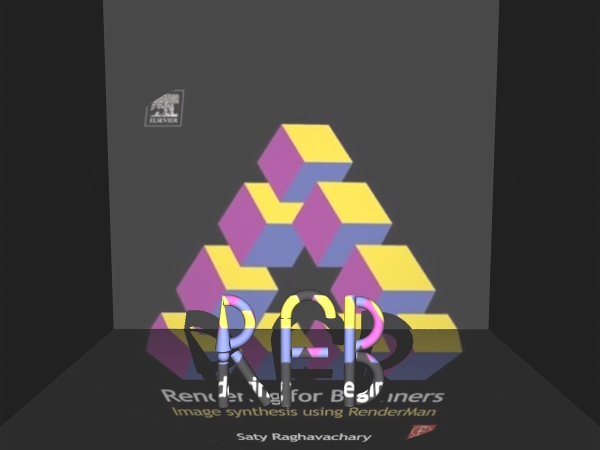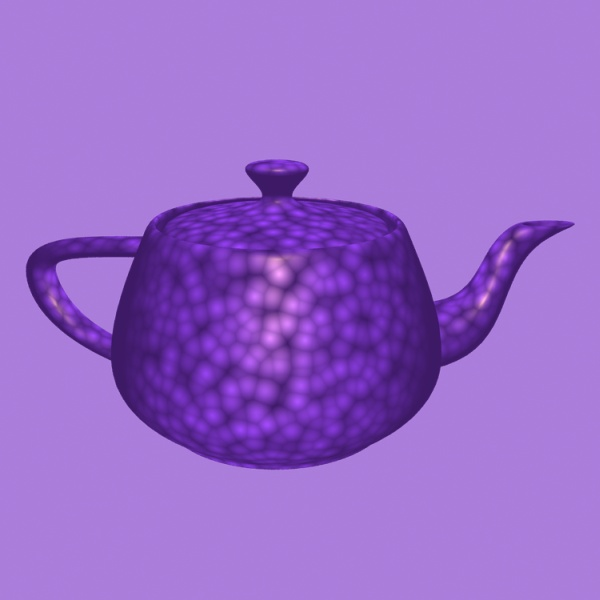Here, the quantity to calculate is P, the micropolygon vert. position. Note that in a surface shader, P is "read only" (can't be altered) whereas here, it is expected to be provided (altered) by the shader.

Note that you need to specify "bounds" in a RIB file where you invoke a displacement shader. The bounds specification tells PRMan how much (maximum) displacement a micropolygon might undergo due to calculations in your shader. A smaller value (for bounds) would lead to 'holes' in the render, while a larger value would lead to waste of memory. See 'App Notes #12, "Using Displacement Shaders"' for more tips. The bounds specification and displacement shader invocation look like this in a RIB file:

# In the following line, 0.2 is a 'spherical' bound for micropoly displacement
Attribute "displacementbound" "coordinatesystem" ["world"] "sphere" [.2]
# displacement shader specified using a 'Displacement' RIB call
Displacement "cloth" "depth" .175 "freq" 300
Surface "LGbrushedmetal" "color basecolor" [ 0.720 0.55 0.145 ] "float Ka" [ .5 ] "float Kd" [ 0.700 ] "float Ks" [ 0.7500 ] "float uroughness" [ 0.500 ] "float vroughness" [ 0.150 ]
# geometry follows..

Three displacement shaders are shown below. Apply them to surfaces in your test RIB files to see their effect.

displacement noisedisp(float freq=1.0, ampl=1.0)
{
normal Nf = normalize( ntransform("shader", N) );

// basic noise
float displ = ampl*(-0.5+noise(PP*freq));

PP += displ*Nf;

P = PP;
N = calculatenormal(P);

}

displacement sinewaves(float freq=1.0, ampl=1.0, sphase=0, tphase=0, paramdir=0)
{
// displace along normal, using sin(s) or sin(t) or both
if(0==paramdir)
{
P += ampl*sin(sphase+s*freq*2*PI)*normalize(N);
}
else if (1==paramdir)
{
P += ampl*sin(tphase+t*freq*2*PI)*normalize(N);
}
else
{
P += ampl*sin(sphase+s*freq*2*PI)*sin(tphase+t*freq*2*PI)*normalize(N);
}
N = calculatenormal(P);
}// sinewaves

/* Copyright notice from the original source: */
/*********************************
*AUTHOR: Ken Musgrave.
*    Conversion to Shading Language and minor modifications by Fredrik Brännbacka.
*
*
* REFERENCES:
*    _Texturing and Modeling: A Procedural Approach_, by David S. Ebert, ed.,
*    F. Kenton Musgrave, Darwyn Peachey, Ken Perlin, and Steven Worley.
*    Academic Press, 1998.  ISBN 0-12-228730-4.
*
*********************************/
#define snoise(x) (2.5*(noise(x)-0.5))

displacement
RMFractalDisp(float freq=1, H = 0.8, lacunarity = 2.5, octaves = 7, offset = 0.9, sharpness = 4, threshold = 12, mfscale=0.5)
{
float result, signal, weight, i, exponent;
normal Nn = normalize(N);
for( i=0; i < octaves; i += 1 ) {
/* First octaves */

if ( i == 0) {
signal = snoise( PP );
if ( signal < 0.0 ) signal = -signal;
signal = offset - signal;
signal = pow( signal, sharpness );
/*This should give you a power function to control
sharpness of the ridges. Or you can just use the
original one -- signal *= signal;*/
result = signal;
weight = 1.0;
}else{

exponent = pow( lacunarity, (-i*H) );
/*
PP.x *= lacunarity;
PP.y *= lacunarity;
PP.z *= lacunarity;
*/
PP = PP * lacunarity;
/* weigh successive contributions by previous signal */
weight = signal * threshold;
weight = clamp(weight,0,1)    ;
signal = snoise( PP );

/* get absolute value of signal*/
signal = abs(signal);

/* invert and translate*/
signal = offset - signal;

/* sharpen the ridge*/
signal = pow( signal, sharpness ); /* Or signal *= signal;*/

/* weight the contribution*/
signal *= weight;
result += signal * exponent;
}
}
result *= mfscale;

P += normalize(N)*result;
N = calculatenormal(P);

}// RMFractalDisp
Use this RIB file to see the RMFractalDisp shader in action.

Note - 3/10/07 - I added a 'freq' parameter to the RMFractalDisp shader above, so please get the above shader again and recompile it (or add the 'freq' parameter to your copy of the shader). If you do that, the RIB file above (download it again, just to be sure) will create the following image:Light shaders are not really "shaders", they are light SOURCES. Here we set Cl, the color of the light. Light shaders let you add to the collection of built-in ambient, point, distant and spot lights that come with PRMan (you can even modify those four).

RIB
rimlt
RIBrainbowlight
RIB
slideprojector [light source]
RIB
TIFF
.tex (MIP map)cellulite
RIB
.dat file